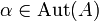# Pseudo-congruent group extensions

## Definition

Suppose$A$ and$B$ are (possibly isomorphic, possibly non-isomorphic groups). Consider two group extensions$G_1, G_2$ both "with normal subgroup$A$ and quotient group$B$." Explicitly, this means we are given two short exact sequences:$1 \to A \to G_1 \to B \to 1$

and:$1 \to A \to G_2 \to B \to 1$

We say that the group extensions are congruent if there is an isomorphism between the short exact sequences. Explicitly, this means that there are automorphisms$\alpha \in \operatorname{Aut}(A)$,$\beta \in \operatorname{Aut}(B)$, and an isomorphism$\varphi: G_1 \to G_2$ such that the following diagram commutes:$\begin{array}{lllll} 1 \to & A \to & G_1 \to & B \to & 1 \\ \downarrow & \downarrow^{\alpha} & \downarrow^{\varphi} & \downarrow^{\beta} & \downarrow\\ 1 \to & A \to & G_2 \to & B \to & 1 \\ \end{array}$

## Related notions

• Congruent group extensions: This is a finer equivalence relation imposed on group extensions, where we require the automorphisms of$A$ and$B$ to both be identity maps.